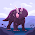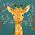## Ileana's Proportion Problems#9   1)  To make lemonade, you need 6 lemons and 8 cups of water. How many lemons do you need if you have 24 cups of water?

Let x be the number of lemons you need if there are 24 cups of water
Word Ratio, Ratio Table, RatioYou need 18 lemons if you have 24 cups of water.#21   2)  3 posters costs \$9.60. At that rate, how many posters can you buy for \$48?

Let x be the number of posters you can buy for \$48
Word Ratio, Ratio Table, RatioAt that rate, you can buy 15 posters.

At Discount Copy, 12 copies cost \$0.66. Melissa needs 56 copies. How much should they cost?

7:50 PM

#### 4 comments :

1.i like your pictures mom!

2.This comment has been removed by the author.

3.the answer for the question is......
let x be the cost of the 56 copies
word ratio, ratio table, find x

cost copies
0.66 12
x 56

12(x) = 0.66(56)
12 x = 36.56/12
x = \$3.08

56 copies cost \$3.08

4.